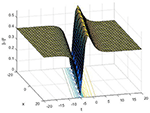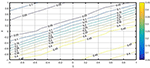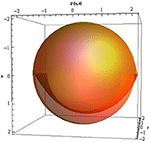Impact Factor 2.638 | CiteScore 2.3
More on impact ›

# Frontiers in Physics## ORIGINAL RESEARCH article

Front. Phys., 14 March 2019 | https://doi.org/10.3389/fphy.2019.00028

# Dark-Bright Optical Soliton and Conserved Vectors to the Biswas-Arshed Equation With Third-Order Dispersions in the Absence of Self-Phase Modulation

• 1Department of Mathematics, Science Faculty, Federal University Dutse, Jigawa, Nigeria
• 2Department of Mathematics, Science Faculty, Firat University, Elazig, Turkey
• 3Department of Mathematics, Cankaya University, Ankara, Turkey
• 4Institute of Space Sciences, Magurele, Romania
• 5Department of Computer Engineering, Istanbul Gelisim University, Istanbul, Turkey

The form-I version of the new celebrated Biswas-Arshed equation is studied in this work with the aid of complex envelope ansatz method. The equation is considered when self-phase is absent and velocity dispersion is negligibly small. New Dark-bright optical soliton solution of the equation emerge from the integration. The acquired solution combines the features of dark and bright solitons in one expression. The solution obtained are not yet reported in the literature. Moreover, we showed that the equation possess conservation laws (Cls).

## 1. Introduction

The study of dynamical systems in non-linear physical models plays an important role in optical fibers, electrical transmission lines, plasma physics, mathematical biology, and many more . This is motivated by the capacity to model the behavior of these systems and other under different physical conditions . These systems are represented by non-linear equations. Seeking the exact solutions of non-linear evolution equations has been an interesting topic in mathematical physics, and the solutions of corresponding models are the ways to well describe their dynamics. Several results have been reported in the last few decades . The main principle for the existence of solitons in metamaterials, optical fibers, and crystals is the existence of a balance between non-linearity and dispersion. It is obvious that some situations may lead to. Recently, Biswas and Arshed  put forward a new model for soliton transmission in optical fibers in the event when self-phase modulation is neglible in the absence of non-linearity.

The third-order model in the absence of self-phase modulation that will be studied in this paper is given by [3, 4]:

The function ψ(x, t) representing the dependent involving t an x which denotes the temporal and spatial components. The first term represents the temporal evolution of the wave, γ represents the(STD) coefficient, α is the coefficient of GVD and σ is the coefficient of the third order dispersion, δ is the coefficient of spatio-temporal 3OD (ST-3OD), Ω is the effect of self-steepening. Finally, μ and θ provide the effect of non-linear dispersion. Dark, bright, combo and singular soliton solutions of Equation (1) have been reported in Biswas and Arshed  and Ekici and Sonmezoglu . But to the best of our knowledge, the dark-bright optical soliton and Cls of the equation have not been reported. In this work, this special solution combining the features of dark and bright optical soliton in one expression will be recovered by applying a suitable ansatz. The Cls of the equation will be derived using the multiplier method [8, 9].

## 2. Dark-Bright Optical Soliton

In order derive the dark-bright soliton solution of the equation, we consider the ansatz solution given by Li et al. :

with

In Equation (2), Ψ denotes the phase shift, k denotes the wave number, ω represents the frequency and ν is the phase constant. We now utilize the ansatz put forward from Li et al. :

where v represents the velocity and η is the pulse width. In the even when λ → 0 or ρ → 0, the Equation (4) transforms to a bright or dark soliton solution. The intensity of A(x, t) is given by

The non-linear phase shift ψNL is represented by

Putting Equation (2) into Equation (1) leads to

Now, putting Equation(4) into Equation(7), expanding the result and equating the combination of coefficients of sech(τ) and tanh(τ), we acquire the independent parametric equations represented by:

Constants:

sech(τ) :

sech2(τ) :

sech3(τ) :

sech4(τ) :

tanh(τ) :

tanh(τ)sech(τ) :

tanh(τ)sech2(τ) :

tanh(τ)sech3(τ) :

tanh2(τ)sech(τ) :

tanh2(τ)sech2(τ) :

tanh3(τ)sech(τ) :

where τ = η(xvt). From the solution of Equations(8)–(19), we observed that β = 0. but, for a dark-bright optical soliton to exist, we require both ρ ≠ 0 and λ ≠ 0. For the sake of compatibility, we considered the case when ρ = λ from the solutions of Equations(8)–(19). We acquire the velocity as

the wave number is represented by

We also acquire the value of δ and α as

The dark-bright optical soliton to the model reads:

and the intensity gives

The phase shift is represented by

The dark-bright soliton Equation (24) represents a soliton combining the features of dark and bright solitons in one expression. The constant β = 0 implies a pronounced “platform” underneath the soliton under non-zero boundary conditions and its asymptotic value approaches λ as |t| → ∞. To analyze the dynamics behavior of the soliton solution Equation (24), we have made numerical evolutions for some perturbations to show the evolution of the dark-bright optical soliton solution. Figures 1-3 shows the profiles surfaces of the dark-bright soliton Equation (24). The obtained soliton Equation (24) possesses the structure of the physical properties of dark and bright optical solitons in the same expression. These solitons appear temporal solitons observed in optical fibers.

FIGURE 1Figure 1. 3D surface of solution Equation(24) by selecting the parameter values of η = 0.1, λ = 0.1, θ = 1, Ω = 0.1.

FIGURE 2Figure 2. The contour surface of solution Equation (24) by selecting the parameter values of η = 0.1, λ = 0.1, θ = 1, Ω = 0.1.

FIGURE 3Figure 3. contour plot in spherical coordinates of solution Equation (24) by selecting the parameter values of η = 0.1, λ = 0.1, θ = 1, Ω = 0.1.

## 3. Conservation Laws

In this part, we will utilize the multiplier to derive the Cls [8, 9]. To achieve this aim, we apply

to transform Equation (1) to a system of PDEs. Putting Equation (27) into Equation (1), we acquire:

Applying the formula for determining equations in , we acquires the multipliers of zeroth-order Λ1(x, t, u, υ), Λ2(x, t, u, υ) for Equation (1)

where c1 is a constant.

1. If c1 = 1 in Equation(29), then we have the following multipliers:

Subsequently, we acquire the fluxes given by:

## 4. Concluding Remarks

In this article, we have explored a suitable ansatz solution to derive a dark-bright soliton solution of the new celebrated Biswas-Arshed equation. observing the solutions derived in Biswas and Arshed  and Ekici and Sonmezoglu , we observed that the solution of the equation acquired in this manuscript is new. The method used here has been proved to be efficient in investigating the combined soliton solution of non-linear models. We finally showed that the equation has conservation laws and we reported the conserved vectors. We hope to apply other techniques to extract additional new forms of solutions of the new model in the future.

## Author Contributions

All authors listed have made a substantial, direct and intellectual contribution to the work, and approved it for publication.

## Funding

This study was Funded by Cankaya University.

## Conflict of Interest Statement

The authors declare that the research was conducted in the absence of any commercial or financial relationships that could be construed as a potential conflict of interest.

## References

1. Whitham GB. Linear and Nonlinear Waves. New York, NY: John Whiley (1974).

2. Agrawal GP. Nonlinear Fiber Optics. New York, NY: Academic Press (1995).

3. Biswas A, Arshed S. Optical solitons in presence of higher order dispersions and absence of self-phase modulation. Optik. (2018) 174:452–9. doi: 10.1016/j.ijleo.2018.08.037

4. Ekici M, Sonmezoglu A. Optical solitons with Biswas-Arshed equation by extended trial function method. Optik. (2018) 177:13–20. doi: 10.1016/j.ijleo.2018.09.134

5. Li Z, Li L, Tian H. New types of solitary wave solutions for the higher order nonlinear Schrödinger equation, Phys Rev Lett. (2000) 84:4096–9. doi: 10.1103/PhysRevLett.84.4096

6. Zhou Q, Zhu Q. Combined optical solitons with parabolic law nonlinearity and spatio-temporal dispersion. J Mod Opt. (2015) 62:483–6. doi: 10.1080/09500340.2014.986549

7. Choudhuri A, Porsezian K. Dark-in-the-Bright solitary wave solution of higher-order nonlinear Schrödinger equation with non-Kerr terms. Opt. Commun. (2012) 285:364–7. doi: 10.1016/j.optcom.2011.09.043.

8. Anco SC, Bluman G. Direct construction method for conservation laws of partial differential equations Part II: general treatment. Eur J Appl Math. (2002) 13:567–85. doi: 10.1017/S0956792501004661

9. Buhe E, Bluman GW. Symmetry reductions, exact solutions, and conservation laws of the generalized Zakharov equations. J Math Phys. (2015) 56:101501. doi: 10.1063/1.4931962

10. Jawad MA, Petkovic MD, Biswas A. Modified simple equation method for nonlinear evolution equations. Appl Math Comput. (2010) 217:869–77. doi: 10.1016/j.amc.2010.06.030

11. Mirzazadeh M, Eslami M, Biswas A. Soliton solutions to KdV6 equation. Nonlinear Dyn. (2015) 80:387–96. doi: 10.1007/s11071-014-1876-1

12. Mirzazadeh M, Mahmood MF, Majid FB, Biswas A, Belic M. Optical solitons in birefringent fibers with riccati equation method. Optoelectron Adv Mat Rapid Commun. (2015) 9:1032–6.

13. Inc M, Aliyu AI, Yusuf A. optical solitons to the nonlinear Schrödinger's equation with Spatio-temporal dispersion using complex amplitude ansatz. J Mod Opt. (2017) 64:2273–80. doi: 10.1080/09500340.2017.1352047

14. Baleanu D, Inc M, Aliyu AI, Yusuf A. Dark optical solitons and conservation laws to the resonance nonlinear Schrödinger equation with Kerr law nonlinearity. Optik. (2017) 147:248–55. doi: 10.1016/j.ijleo.2017.08.080

15. Inc M, Aliyu AI, Yusuf A. Solitons and conservation laws to the resonance nonlinear Schrödinger equation with both spatio-temporal and inter-modal dispersions. Optik. (2017) 142:509–22. doi: 10.1016/j.ijleo.2017.06.010

16. Inc M, Hashemi MS, Aliyu AI. Exact solutions and conservation laws of the Bogoyavlenskii equation, Acta Phys Polon A. (2018) 133:1133–7. doi: 10.12693/APhysPolA.133.1133

17. Aliyu AI, Inc M, Yusuf A, Baleanu D. Symmetry analysis, explicit solutions, and conservation laws of a sixth-order nonlinear ramani equation. Symmetry. (2018) 10:341. doi: 10.3390/sym10080341

18. Inc M, Aliyu AI, Yusuf A, Baleanu D. Novel optical solitary waves and modulation instability analysis for the coupled nonlinear Schrödinger equation in monomode step-index optical fibers. Superlattices Microstruct. (2018) 113:745–53. doi: 10.1016/j.spmi.2017.12.010

19. Inc M, Aliyu AI, Yusuf A, Baleanu D. Optical solitons, conservation laws and modulation instability analysis for the modified nonlinear Schrödinger's equation for Davydov solitons. J Electromagn Waves Appl. (2018) 32:858–73. doi: 10.1080/09205071.2017.1408499

20. Inc M, Aliyu AI, Yusuf A, Baleanu D. Optical solitary waves, conservation laws and modulation instability analysis to the nonlinear Schrödinger's equation in compressional dispersive Alven waves. Optik. (2017) 155:257–66. doi: 10.1016/j.ijleo.2017.10.109

CrossRef Full Text

21. Zhou Q, Mirzazadeh M, Ekici M, Sonmezoglu A. Analytical study of solitons in non-Kerr nonlinear negative-index materials. Nonlinear Dyn. (2016) 86:623–38. doi: 10.1007/s11071-016-2911-1

22. Zhou Q. Analytical solutions and modulation instability analysis to the perturbed nonlinear Schrödinger equation. J Mod Opt. (2014) 61:500–3. doi: 10.1080/09500340.2014.897391

CrossRef Full Text

23. Taghizadeh N, Mirzazadeh M, Farahrooz F. Exact solutions of the nonlinear Schrodinger equation by the first integral method. J Math Anal Appl. (2011) 374:549–53. doi: 10.1016/j.jmaa.2010.08.050

24. Inc M, Aliyu AI, Yusuf A, Baleanu D. Combined optical solitary waves and conservation laws for nonlinear Chen-Lee-Liu equation in optical fibers. Optik. (2018) 158:297–304. doi: 10.1016/j.ijleo.2017.12.075

Keywords: complex envelope ansatz, dark-bright optical soliton, Biswas-Arshed equation, conserved vectors, multiplier, numerical simulations

Citation: Aliyu AI, Inc M, Yusuf A, Baleanu D and Bayram M (2019) Dark-Bright Optical Soliton and Conserved Vectors to the Biswas-Arshed Equation With Third-Order Dispersions in the Absence of Self-Phase Modulation. Front. Phys. 7:28. doi: 10.3389/fphy.2019.00028

Received: 04 December 2018; Accepted: 18 February 2019;
Published: 14 March 2019.

Edited by:

Jesus Martin-Vaquero, University of Salamanca, Spain

Reviewed by:

Emrullah Yasar, Uludaǧ University, Turkey
Haci Mehmet Baskonus, Harran University, Turkey

Copyright © 2019 Aliyu, Inc, Yusuf, Baleanu and Bayram. This is an open-access article distributed under the terms of the Creative Commons Attribution License (CC BY). The use, distribution or reproduction in other forums is permitted, provided the original author(s) and the copyright owner(s) are credited and that the original publication in this journal is cited, in accordance with accepted academic practice. No use, distribution or reproduction is permitted which does not comply with these terms.

*Correspondence: Mustafa Inc, minc@firat.edu.tr